### Home > A2C > Chapter 3 > Lesson 3.2.3 > Problem3-127

3-127.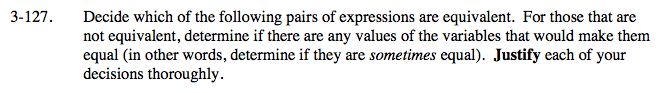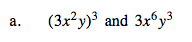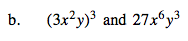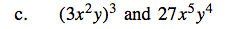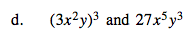First, remember that the 3 is also being cubed.

Remember what happens when a squared variable is being put to a power.

Only the expressions in part (b) are equivalent.

Think about numbers like 0 and 1.

The expressions in part (a) are equivalent only when x = 0 or y = 0.
The expressions in part (c) are equivalent when both x and y are 1 or when one of the variables is 0.